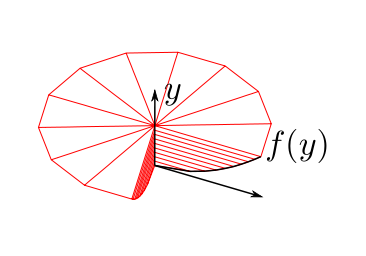# Multiple Dimensions - Extending Disk IntegrationIn calculus, disk integration, allows us to calculate the volume of a solid by integrating along an axis. It models the resulting shape as a stack of disks of infinitesimal thickness. Most of the time, we chose an axis of revolution, which results in a circular disk, though this is not necessary. The washer method uses the same idea, and gives us a 'hollow' solid of revolution. We'd push this idea even further.

Let's consider the case of a circle with radius $r$. We know that it has an area of $\pi r^2$ and a perimeter of $2 \pi r$, but how can we show this?Wiki

One basic approach, is to say that the circle is the set of points such that $x^2 + y^2 \leq r^2$, and hence we want to calculate $\int_R 1, dR$. Let's use the area element, with a chosen axis of the $x -$axis. When $x = X$, we see that $y^2 \leq r^2 - X^2$ and so $- \sqrt{ r^2 - X^2} \leq y \leq \sqrt{ r^2 - X^2}$. Hence, at $x = X$, the area contributed is equal to $\sqrt{ r^2 - X^2} - (-\sqrt{ r^2 - X^2} ) = 2 \sqrt{ r^2 - X^2}$. As such, this allows us to calculate that:

$\int A(x) dx = \int_{-r}^r 2 \sqrt{ r^2 - x^2} \, dx = \pi r^2$Wiki

Another way to use the area element, is to think about obtaining the area of the circle, by considering how faraway each of the points are. In this case, our chosen axis would be the radius of the circle. When the radius is $R$, the contribution to the area is $p(R)$, which denotes the perimeter of the circle. This tells us that

$\int_0^r P(R) \, dR = \pi r^2.$

Applying the fundamental theorem of calculus, we get that $P(r) = 2 \pi r$. We see this relationship represented in the previous 2 problems, where we worked on the 2 dimensional circle and the 3 dimensional sphere.

This seems like a torturous way to obtain the area and perimeter of a circle, which are already well known. In the following questions, we will apply this method to find the area and perimeter of a sphere in 4 dimensions!

###### Image credit: Wikipedia Macks, Wikipedia KsmrqNote by Calvin Lin
7 years, 2 months ago

This discussion board is a place to discuss our Daily Challenges and the math and science related to those challenges. Explanations are more than just a solution — they should explain the steps and thinking strategies that you used to obtain the solution. Comments should further the discussion of math and science.

When posting on Brilliant:

• Use the emojis to react to an explanation, whether you're congratulating a job well done , or just really confused .
• Ask specific questions about the challenge or the steps in somebody's explanation. Well-posed questions can add a lot to the discussion, but posting "I don't understand!" doesn't help anyone.
• Try to contribute something new to the discussion, whether it is an extension, generalization or other idea related to the challenge.

MarkdownAppears as
*italics* or _italics_ italics
**bold** or __bold__ bold
- bulleted- list
• bulleted
• list
1. numbered2. list
1. numbered
2. list
Note: you must add a full line of space before and after lists for them to show up correctly
paragraph 1paragraph 2

paragraph 1

paragraph 2

[example link](https://brilliant.org)example link
> This is a quote
This is a quote
    # I indented these lines
# 4 spaces, and now they show
# up as a code block.

print "hello world"
# I indented these lines
# 4 spaces, and now they show
# up as a code block.

print "hello world"
MathAppears as
Remember to wrap math in $$ ... $$ or $ ... $ to ensure proper formatting.
2 \times 3 $2 \times 3$
2^{34} $2^{34}$
a_{i-1} $a_{i-1}$
\frac{2}{3} $\frac{2}{3}$
\sqrt{2} $\sqrt{2}$
\sum_{i=1}^3 $\sum_{i=1}^3$
\sin \theta $\sin \theta$
\boxed{123} $\boxed{123}$

Sort by:

Thanks for a nice presentation. What about the hollow cylinder method ?

- 7 years, 2 months ago

Yes, there are also extensions to the shell method. I avoided talking about it, because it's not intuitive and most people have some trouble grasping it. It uses the same idea of "area element", which is how I prefer to think about it.

Staff - 7 years, 2 months ago

Thank you. In some case the integral is simple by one method or the other.

- 7 years, 2 months ago

0/3

- 6 years, 10 months ago

Nice presentation

- 7 years, 1 month ago

i dont know

- 7 years, 1 month ago